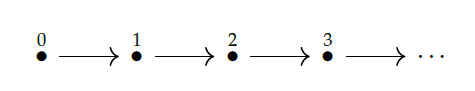So far the only _examples_ of posets I've talked about in the lectures are the real numbers \$$\mathbb{R}\$$ and the natural numbers \$$\mathbb{N}\$$ with their usual order \$$\le\$$. Of course every natural number is a real number, so there's a function

$i : \mathbb{N} \to \mathbb{R}$

sending any natural number \$$x \in \mathbb{N}\$$ to the exact same number regarded as a real number. This function is monotone, so you now know instinctively to ask this question:

**Puzzle 21.** Does the monotone function \$$i : \mathbb{N} \to \mathbb{R}\$$ have a left adjoint? Does it have a right adjoint? If so, what are they?

This is nice, but we need to look at other examples to appreciate the diversity of posets. Both \$$\mathbb{N}\$$ and \$$\mathbb{Z}\$$ have a very special property. They are **[totally ordered sets](https://en.wikipedia.org/wiki/Total_order)**: posets such that

$\textrm{ for all } x, y, \textrm{ either } x \le y \textrm{ or } y \le x .$

If you want to show off, you can call totally ordered sets **tosets**. They're also called **linearly ordered**, because you can imagine them as lines:Totally ordered sets are limiting. Suppose you're trying to order foods on a restaurant menu based on how much you like them. What's better: a cheese sandwich or a pancake? There may be no answer, because you like them in _different ways_. To get a totally ordered set you have to ignore this and arrange all the foods in a line.

In standard economics we _do_ try to arrange everything in a line. We measure the worth of everything in real numbers: numbers of _dollars_. There's even a theorem to justify this, proved by [von Neumann and Morgenstern](https://en.wikipedia.org/wiki/Von_Neumann%E2%80%93Morgenstern_utility_theorem). But the assumptions of this theorem don't hold in real life. It's mainly just _convenient_ to measure value, or "utility", in real numbers. With computer technologies we could set up cryptocurrencies based on other posets. But will we?

Luckily, human thought as a whole is not limited to total orders. A good example is logic. Logic, in its simplest form, is about statements \$$P, Q, R, \dots \$$ and whether one statement implies another. If \$$P\$$ implies \$$Q\$$ we often write \$$P \implies Q\$$. There are many kinds of logic, but every kind I know, this relation \$$\implies\$$ makes statements into a preorder, since we have

1) reflexivity: \$$P \implies P\$$

2) transitivity: if \$$P \implies Q\$$ and \$$Q \implies R \$$ then \$$P \implies R\$$.

Often people make this preorder into a poset by imposing this rule:

3) antisymmetry: if \$$P \implies Q\$$ and \$$Q \implies P\$$ then \$$P = Q \$$.

This amounts to decreeing that we count two statements as "the same" if they both imply each other. We may not always want to do this. And we certainly don't want a linear order: it's easy to find examples of statements such that neither \$$P \implies Q\$$ nor \$$Q \implies P\$$, like "I am a millionaire" and "I am happy", or "I like this food for breakfast" and "I like this food for lunch".

So, to continue our study of preorders, posets, monotone functions and Galois connections, we'll turn to logic! Category-theoretic logic is an enormous wonderful field, but we'll just do a bit of logic based on the poset of subsets of a set, followed by a bit of logic based on the poset of partitions of a set. The latter underlies Fong and Spivak's discussion of "generative effects" in Chapter 1.

**[To read other lectures go here.](http://www.azimuthproject.org/azimuth/show/Applied+Category+Theory#Course)**